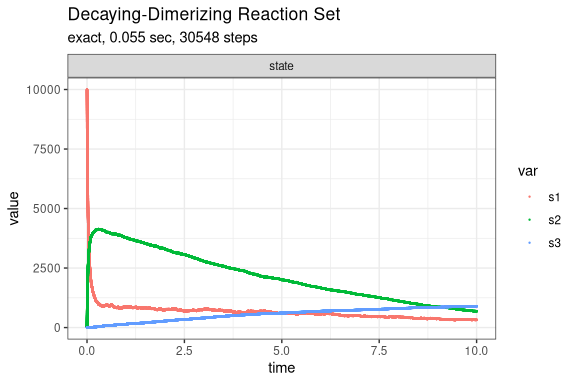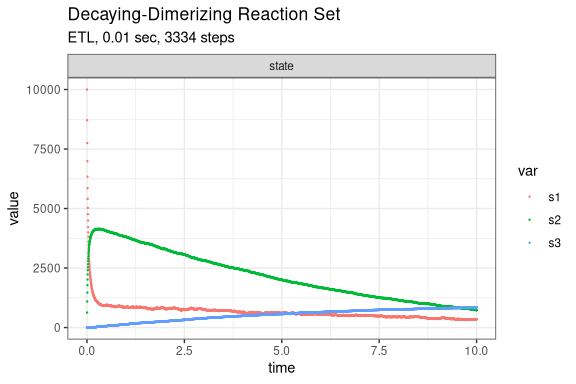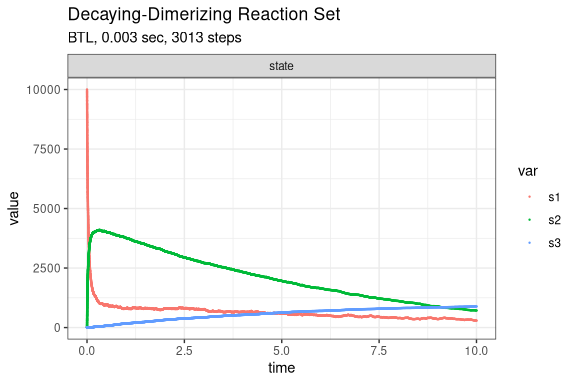# Decaying-Dimerization Reaction Set

The Decaying-Dimerization Reaction Set (Gillespie 2001) consists of three species and four reaction channels.

      S1 --c1--> 0
S1 + S1 --c2--> S2
S2 --c3--> S1 + S1
S2 --c4--> S3

Define parameters

library(GillespieSSA2)
sim_name <- "Decaying-Dimerizing Reaction Set"
final_time <- 10
params <- c(c1 = 1.0, c2 = 0.002, c3 = 0.5, c4 = 0.04)
initial_state <- c(s1 = 10000, s2 = 0, s3 = 0)

Define reactions

reactions <- list(
reaction("c1 * s1",        c(s1 = -1)),
reaction("c2 * s1 * s1",   c(s1 = -2, s2 = +1)),
reaction("c3 * s2",        c(s1 = +2, s2 = -1)),
reaction("c4 * s2",        c(s2 = -1, s3 = +1))
)

Run simulations with the Exact method

set.seed(1)
out <- ssa(
initial_state = initial_state,
reactions = reactions,
params = params,
final_time = final_time,
method = ssa_exact(),
sim_name = sim_name
)
plot_ssa(out)Run simulations with the Explict tau-leap method

set.seed(1)
out <- ssa(
initial_state = initial_state,
reactions = reactions,
params = params,
final_time = final_time,
method = ssa_etl(tau = 0.003),
sim_name = sim_name
)
plot_ssa(out)Run simulations with the Binomial tau-leap method

set.seed(1)
out <- ssa(
initial_state = initial_state,
reactions = reactions,
params = params,
final_time = final_time,
method = ssa_btl(),
sim_name = sim_name
)
plot_ssa(out)